×
Get Full Access to MSU - AEC 2713 - Class Notes - Week 14
Get Full Access to MSU - AEC 2713 - Class Notes - Week 14

×

MSU / Agricultural Economics / AEC 2713 / What is the perfectly competitive market?

# What is the perfectly competitive market? Description

##### Description: chapter 14 notes
28 Pages 262 Views 0 Unlocks
Reviews

## How is it related to total and averagrevenue?## What is marginal revenue?## • What is a perfectly competitive market?Econ.14 Tuesday,  November  15, 2016 11:08 AMChapter 14: Firms in competitive markets Chapter Overview: • What is a perfectly competitive market?  • What is marginal revenue? How is it related to total and averagrevenue? • How does a firm in a competitive market determine the quantity  that maximizes profits? • When might a competitive firm shut dowDon't forget about the age old question of greco roman household codes
We also discuss several other topics like Who was the sister of Meleagros?
We also discuss several other topics like What is the region of polar rectangle?
We also discuss several other topics like How do we calculate entropy by using the Boltzmann hypothesis?
If you want to learn more check out Decribe the structure of DNA inside the nucleus.
We also discuss several other topics like e) What events are celebrated and highlighted and how they are celebrated f) Where do org cultures come from?
n in the short run? • When might a competitive firm shut down in the long run? • What does the market supply curve look like in the short run? Inthe long run? Scenario: starting your own business: • Three years after graduating, you are running your own business • You must decide how much to produce, what price to charge, how  many workers to hire, etc. • What factors should affect these decisions? ○ Your costs (covered in Chapter 13) ○ How much competition you face • Look at how firms behave in perfectly competitive markets Charcteristics of Perfect Competition: Many buyers and sellers The goods offered for sale are largely the same Firms can freely enter or exit the market • Because of 1 & 2, each buyer and seller is a “price taker” • These firms do not set their price; they take the price as given • Example – a farmer does not set the price for the commodity cornthat is sold e           • Because of 1 & 2, each buyer and seller is a “price taker” • These firms do not set their price; they take the price as given • Example – a farmer does not set the price for the commodity cornthat is sold Revenue of a competitive Firm Total revenue (TR) = price x quantity Average revenue (AR) = total revenue/ quantity = price Marginal revenue (MR) = change in total revenue/ change in  quantity = price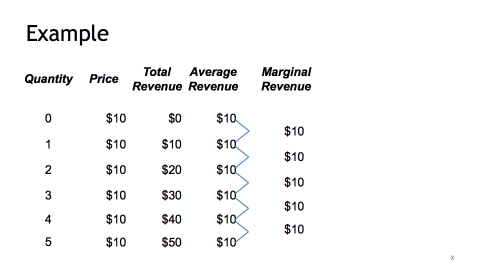MR = price for a competitive firm • A competitive firm can keep increasing its output without  affecting the market price ○ It doesn’t matter how many bushels of corn that a farmer  sells, the market price for corn will not change • So, each one-unit increase in Quantity causes revenue to rise byP (MR = P) • MR = P is only true for firms in competitive markets Profit Maximization • What Q maximizes the firm’s profits? • To determine the answer, “think at the margin” If Q increases by one unit revenue rises by MR cost rises by MC • To determine the answer, “think at the margin” If Q increases by one unit revenue rises by MR cost rises by MC • If MR > MC, then increase Q to raise profit • If MR < MC, then reduce Q to raise profit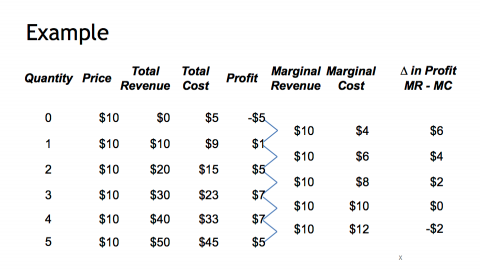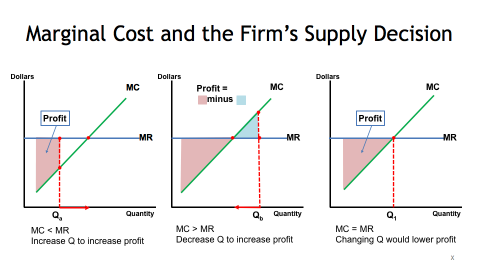Marginal Cost and the firm's supply decision •If the price rises to P2, then the profit maximizing quantity  rises to Q2. Marginal Cost and the firm's supply decision • If the price rises to P2, then the profit maximizing quantity  rises to Q2. • The MC curve determines the firm’s Q at any price. • Hence, the MC curve is the firm’s supply curve.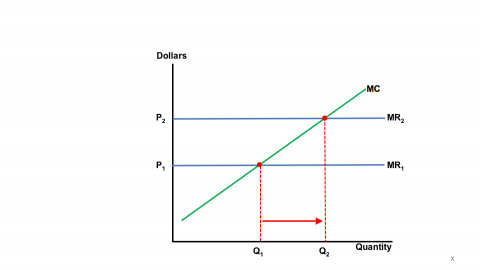Shutdown versus Exit • Shutdown • ○ Exit A short-run decision not to produce anything because of  market conditions. ○ A long-run decision to leave the market •Long run phenA key difference ○ If the firm is shutdown in the short run, the firm must stilpay Fixed Costs ○ If the firm exits in the long run, there are zero costs Short run  phenomenon menonlThe irrelevance of sunk costs • Sunk cost: accost that has already been committed and cannot be  recovered • Sunk costs should be irrelevant to decisions; you must pay them  regardless of your choice • Fixed Costs are sunk costs. The firm must pay its Fixed Costs  whether it produces or shuts down. •So, Fixed Costs should not matter in the decision of whether to  shut down.      . • So, Fixed Costs should not matter in the decision of whether to  shut down.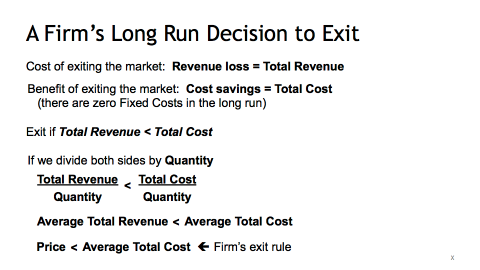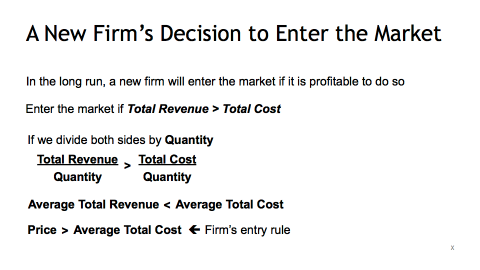A competitive Firm's Supply Curve • Determine this firm’s total profit • Identify the area on the graph that represents the firm’s profit.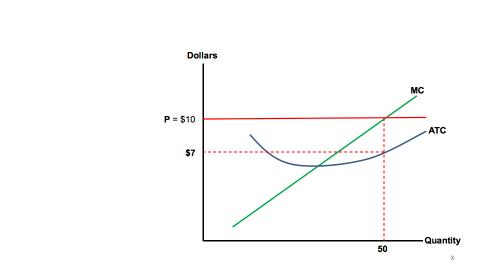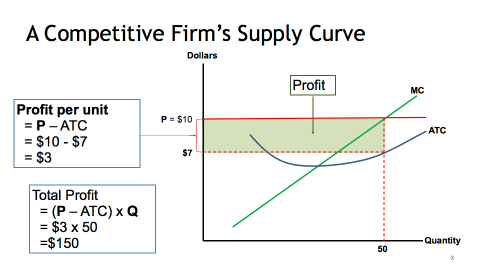• Determine this firm’s total loss, assuming AVC < \$3 • Identify the area on the graph that represents the firm’s loss. .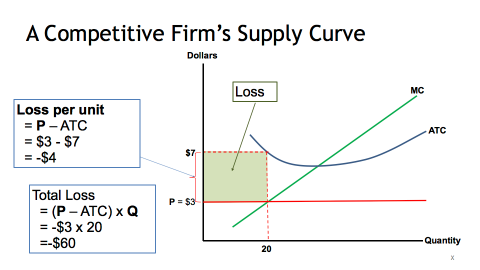Market Supply: Assumptions 1. All existing firms and potential entrants have identical  costs a. This is a simplistic assumption; in the real world, thiassumption does not strictly hold 2. Each firm’s costs do not change as other firms enter or exitthe market a. This is a simplistic assumption; in the real world, thiassumption does not strictly hold 3.The number of firms in the market is a. Fixed in the short run due to fixed costs b. Variable in the long run due to free entry and exit      assumption does not strictly hold 3. The number of firms in the market is a. Fixed in the short run due to fixed costs b. Variable in the long run due to free entry and exit The short run market supply curve • As long as P ≥ AVC, each firm will produce its profit-maximizinquantity, where MR = MC • Recall from Chapter 4: At each price, the market quantity supplied is the sum of  quantities supplied by all firms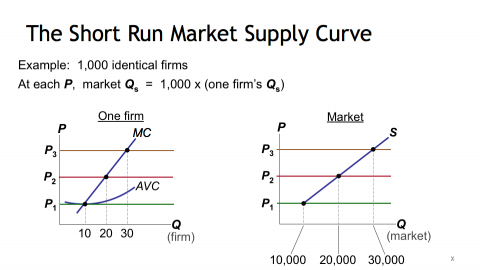Entry and exit in the long run • In the Long Run, the number of firms can change due to entry &  exit • If existing firms earn positive economic profit, ○ New firms enter, Short Run market supply shifts right ○ P falls, reducing profits and slowing entry • If existing firms incur losses ○ Some firms exit, Short Run market supply shifts left ○ P rises, reducing remaining firms’ losses The zero-profit condition • Long run equilibrium – ○ ome rms ex, or un mare suppy ss e ○ P rises, reducing remaining firms’ losses The zero-profit condition • Long run equilibrium The process of entry or exit is complete – remaining firms earn  zero economic profit • Zero economic profit occurs when P = ATC • Since firms produce where P = MR = MC, the zero economic profit condition is P = MC = ATC • Recall that MC intersects ATC at minimum ATC • Hence, in the long run, P = minimum ATC Why do firms stay in business in profit = 0? • •Recall that economic profit is revenue minus all costs (explicitcosts plus implicit costs). Implicit costs include the  opportunity cost of the owner’s time and money. In the zero economic profit equilibrium, ○ Firms earn enough revenue to cover these costs ○ Accounting profit is positive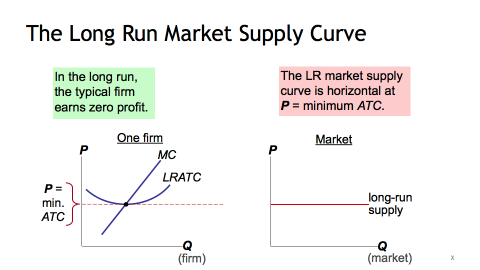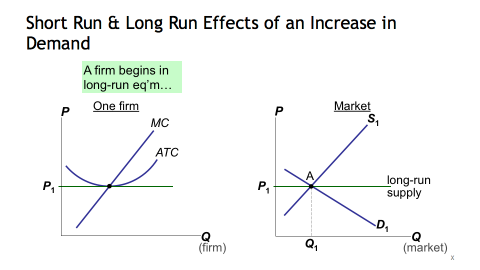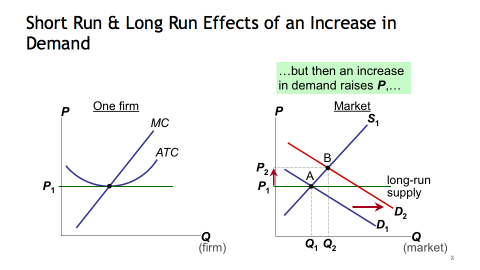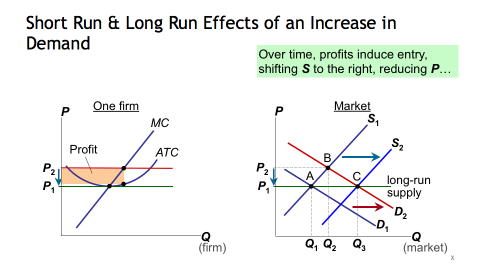WONT HAVE TO  DRAW JUST  EXPLAINWhy the LR supply curve might slope upward •Why the LR supply curve might slope upward • The long run market supply curve is horizontal if: ○ All firms have identical costs and ○ Costs do not change as other firms enter or exit the market • If either of these assumptions is not true, then the long run  supply curve slopes upward Why the LR Supply Curve Might Slope Upward Firms have different costs NOT TESTING ON TH• As P rises, firms with lower costs enter the market before thosewith higher costs • Further increases in P make it worthwhile for higher-cost firms  to enter the market, which increases the quantity supplied • Therefore, the LR market supply curve will slope upward • At any P, ○ For the marginal firm, P = minimum ATC and profit = 0 ○ For lower-cost firms, profit > 0 Why the LR Supply Curve Might Slope Upward Costs rise as firms enter the market NOT TESTING ON TH• In some industries, the supply of a key input is limited (e.g., the amount of land suitable for farming is fixed) • The entry of new firms increases demand for this input, causing its price to rise • This increases the cost for all firms • Therefore, an increase in P is required to increase the market  quantity supplied, so the supply curve is upward sloping Conclusion The efficiency of a competitive market • Recall that MC is the cost of producing the marginal unit. • P is the value to the buyers of the marginal unit • IS  IS           . • P is the value to the buyers of the marginal unit •

Page ExpiredIt looks like your free minutes have expired! Lucky for you we have all the content you need, just sign up here